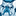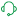Announcements
Applications are open for the 2024 Qlik Luminary Program. Apply by December 15 here.
cancel
Showing results for
Did you mean:Contributor III

## Repeating Dimension Values

Hello

I would like to suppress a dimension value if the value above is the same value.  I want to do this in a straight table.

Thanks

Frank

Labels (2)

• ### dimension

1 Solution

Accepted SolutionsSupport

Hello,

With Qlik Sense you might be able to do it by using set analysis.

You could try the below:

1. Create a new calculated field that checks if the current dimension value is the same as the previous dimension value:

if(Peek(FieldName) = FieldName, 0, 1) as Display

1. Use this calculated field as a dimension in your straight table:

Dimension: Display

1. In the expression for the column in your straight table, use set analysis to only display values where Display is 1:

Sum({<Display = {1}>} [Measure Field])

This should suppress repeating dimension values in your straight table, only displaying unique values. You can adjust the logic in the calculated field as needed based on your data and requirements.Support

Hello,

With Qlik Sense you might be able to do it by using set analysis.

You could try the below:

1. Create a new calculated field that checks if the current dimension value is the same as the previous dimension value:

if(Peek(FieldName) = FieldName, 0, 1) as Display

1. Use this calculated field as a dimension in your straight table:

Dimension: Display

1. In the expression for the column in your straight table, use set analysis to only display values where Display is 1:

Sum({<Display = {1}>} [Measure Field])

This should suppress repeating dimension values in your straight table, only displaying unique values. You can adjust the logic in the calculated field as needed based on your data and requirements.Tags
Community Browser